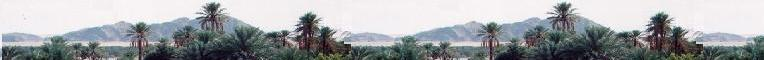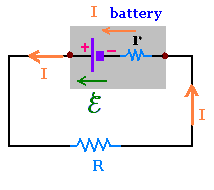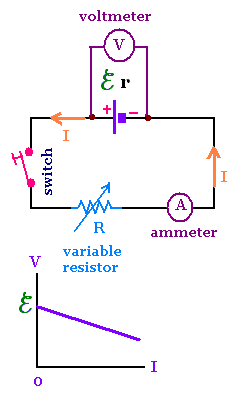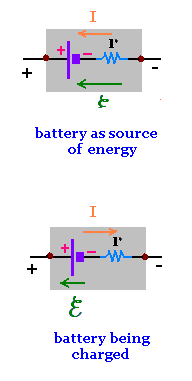Electrostatics

Electromagnetics

Electricity &
Magnetism

# DC circuits

An electric current crossing a circuit can be a direct-current DC, or an alternating-current AC. The sense of the dc-current is continuous along one direction, whereas, the sense of the ac-current oscillates back and forth.

### 1. Sources of electromotive force emf

To have a current in an electric circuit, This circuit must be supplied by an electric energy source.

The continuous current or dc-current exists in a circuit if the circuit contains the element called a source of electromotive force emf, which is the source of electric energy. This source of emf provides electric energy to the charge carriers to move around a circuit.

The electric energy sources include batteries, generators, thermocouples, and solar cells. These devices transform energy from some other form to electric energy. A battery transforms chemical energy to electric energy, a generator from mechanical energy to electric energy, and solar cell from electromagnetic radiation energy (light energy) to electric energy.

In this chapter, we are interested in the continuous electric current (dc), and the energy from a battery.

### 2. Potential difference in a battery

A battery is a source of emf. When the two terminals + and - of the battery are connected to a resistor, the battery establishes a current in the circuit. For some time, the current in the circuit becomes steady, thus the battery maintains a constant potential difference across its terminals: the positive terminal at the higher potential (+) and the negative terminal at the lower potential (-).Consider a battery of emf ℰ and internal resistance r that supplies a resistor R. The sense of the current I, across the resistor R, is from the positive terminal to the negative terminal , and from the the negative terminal to the positive terminal inside the battery.

The potential difference across the battery is equal to :

V = ℰ - rI

The sign - comes from the fact that the current circulates within the battery in the opposite sense
.

Potential difference across the battery of
emf ℰ and internal resistance r:

V = ℰ - rIThe two important characteristics of a battery are its electromotive force ℰ and its internal resistance. The emf characterizes the energy that the battery provides the charge carriers, and the internal resistance is the battery's own resistance. These two characteristics are constant.

To vary V, we vary I by changing the value of the variable resistor. Therefor we define the emf ℰ of the battery as the potential difference across the battery when the current is null (switch in circuit is open). We can measure the emf ℰ by placing a voltmeter in parallel with the battery with the switch open. With an ammeter in series with the the variable resistor, and the switch closed, we measure the internal resistance r: r = (ℰ - V)/I . V is the battery's terminal potential difference read by the voltmeter, and I is the current read by the ammeter.

The emf is a voltage, that gives for the battery a polarity. Hence the terminals are distinguishable, and the sense of the battery's emf is from its negative terminal to its positive terminal.

### 3. Terminal potential difference across the battery

The emf ℰ of a battery has the same dimension as electric potential, hence the dimension of energy per unit charge. The emf of a battery is its electric potential energy per unit charge. This energy is given to the charge carriers to move from a terminal to another without any electric field, but only by chemical process. Hence the name electromotive force. The potential difference V of a battery is generally equal to its emf because its internal resistance is small and can be neglected (the 12-V automobile battery has a resistance around 5mΩ).If the sense of the current in a battery is the same as the sense of its emf, the battery is discharging.

If the sense of the current in a battery is opposite the sense of its emf, the battery is charging. In this case the terminal potential difference across the battery is equal to
V = ℰ + rI
(the 12-V automobile battery, in good conditions, being charged must reads about 14V).

 chimie labs | Physics and Measurements | Probability & Statistics | Combinatorics - Probability | Chimie | Optics | contact |